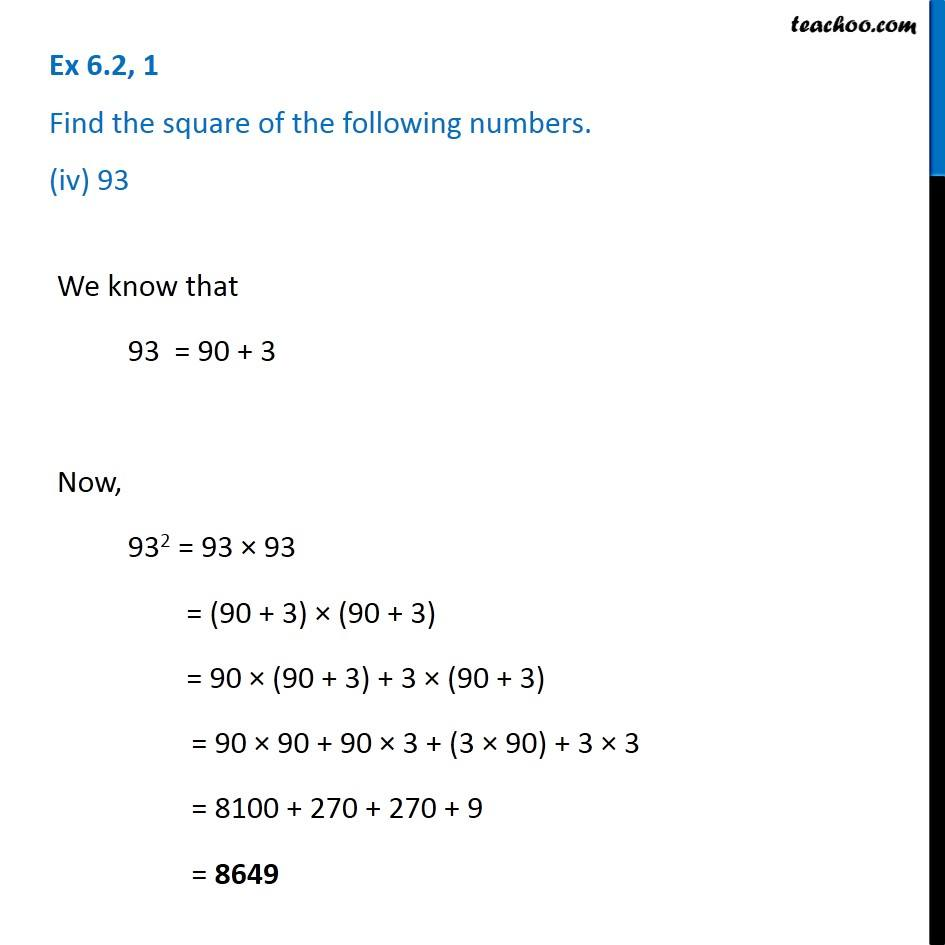Ex 6.2

Chapter 6 Class 8 Squares and Square Roots
Serial order wiseGet live Maths 1-on-1 Classs - Class 6 to 12

### Transcript

Ex 6.2, 1 Find the square of the following numbers. (iv) 93We know that 93 = 90 + 3 Now, 932 = 93 × 93 = (90 + 3) × (90 + 3) = 90 × (90 + 3) + 3 × (90 + 3) = 90 × 90 + 90 × 3 + (3 × 90) + 3 × 3 = 8100 + 270 + 270 + 9 = 8649